# A stake is pulled out of the ground by means of two ropes as shown. Knowing that the tension in one rope is 120 N, determine by trigonometry the magnitude and direction of the force P so that the resultant is a vertical force of 160 N.

Question-AnswerCategory: Engineering MechanicsA stake is pulled out of the ground by means of two ropes as shown. Knowing that the tension in one rope is 120 N, determine by trigonometry the magnitude and direction of the force P so that the resultant is a vertical force of 160 N.

A stake is pulled out of the ground by means of two ropes as shown. Knowing that the tension in one rope is 120 N, determine by trigonometry the magnitude and direction of the force P so that the resultant is a vertical force of 160 N.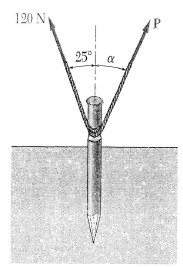Step: 1

A stake is pulled out of the ground by means of two ropes.
The tension in one rope,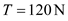The resultant force,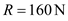Resultant is a vertical force.
Hence,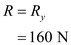The horizontal component of the resultant force is zero,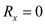Step: 2

Diagram of the force system: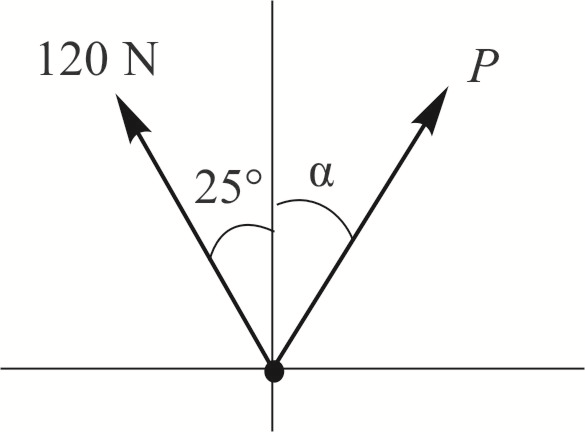Step: 3

The horizontal component of the resultant force is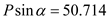…… (1)
The vertical component of the resultant force is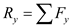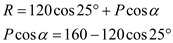…… (2)

Step: 4

Dividing the equation (1) with equation (2),Therefore, the direction of the force P isStep: 5

From the equation (1),Therefore, the magnitude of the force P is